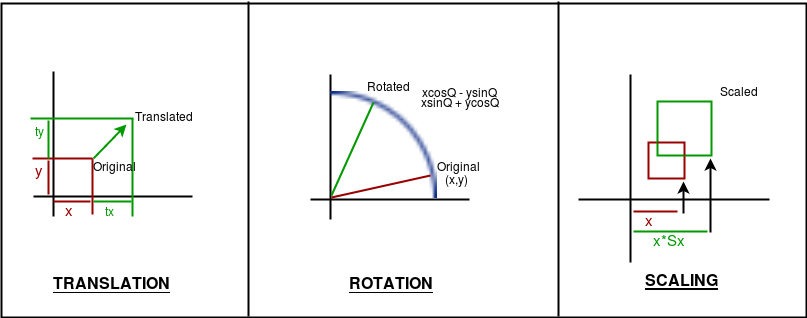# Basic Transformations in OPENGL

• Difficulty Level : Basic
• Last Updated : 08 Jul, 2022

Transformations play a very important role in manipulating objects on the screen. It should be noted that here the algorithms will be implemented in code and the built-in functions will not be used to give a good understanding of how the algorithms work. Also, note that all transformations are implemented in 2D. There are three basic kinds of Transformations in Computer Graphics: 1. Translation 2. Rotation 3. ScalingAlgorithms:

1. Translation: Translation refers to moving an object to a different position on the screen.

```Formula:  X = x + tx
Y = y + ty
where tx and ty are translation coordinates

The OpenGL function is glTranslatef( tx, ty, tz );```

2. Rotation: Rotation refers to rotating a point.

```Formula:  X = xcosA - ysinA
Y = xsinA + ycosA,
A is the angle of rotation.
The above formula will rotate the point around the origin.
To rotate around a different point, the formula:
X = cx + (x-cx)*cosA - (y-cy)*sinA,
Y = cy + (x-cx)*sinA + (y-cy)*cosA,
cx, cy is centre coordinates,
A is the angle of rotation.

The OpenGL function is glRotatef (A, x, y, z). ```

3. Scaling: Scaling refers to zooming in and out an object on different scales across axes.

```Formula: X = x*sx
Y = y*sy,    sx, sy being scaling factors.

The OpenGL function is glScalef(float x, float y, float z)```

Note: If combined transformations are to be applied, follow the order: translate, rotate, scale

Implementation:

## C

 `// C code to implement basic ` `// transformations in OPENGL ` `#include ` `#include ` `#include ` `#include ` ` `  `// Window size ` `#define maxWD 640 ` `#define maxHT 480 ` ` `  `// Rotation speed ` `#define thetaSpeed 0.05 ` ` `  `// This creates delay between  ` `// two actions ` `void` `delay(unsigned ``int` `mseconds) ` `{ ` `    ``clock_t` `goal = mseconds + ``clock``(); ` `    ``while` `(goal > ``clock``()); ` `} ` ` `  `// This is a basic init for the  ` `// glut window ` `void` `myInit(``void``) ` `{ ` `    ``glClearColor(1.0, 1.0,  ` `                 ``1.0, 0.0); ` `    ``glMatrixMode(GL_PROJECTION); ` `    ``glLoadIdentity(); ` `    ``gluOrtho2D(0.0, maxWD,  ` `               ``0.0, maxHT); ` `    ``glClear(GL_COLOR_BUFFER_BIT); ` `    ``glFlush(); ` `} ` ` `  `// This function just draws  ` `// a point ` `void` `drawPoint(``int` `x, ``int` `y) ` `{ ` `    ``glPointSize(7.0); ` `    ``glColor3f(0.0f, 0.0f, 1.0f); ` `    ``glBegin(GL_POINTS); ` `    ``glVertex2i(x, y); ` `    ``glEnd(); ` `} ` ` `  `void` `rotateAroundPt(``int` `px, ``int` `py,  ` `                    ``int` `cx, ``int` `cy) ` `{ ` `    ``float` `theta = 0.0; ` `    ``while` `(1)  ` `    ``{ ` `        ``glClear(GL_COLOR_BUFFER_BIT); ` `        ``int` `xf, yf; ` ` `  `        ``// Update theta anticlockwise  ` `        ``// rotation ` `        ``theta = theta + thetaSpeed; ` ` `  `        ``// Check overflow ` `        ``if` `(theta>= (2.0 * 3.14159)) ` `            ``theta = theta - (2.0 * 3.14159); ` ` `  `        ``// actual calculations.. ` `        ``xf = (cx + (``int``)((``float``)(px - cx) *  ` `              ``cos``(theta)) - ((``float``)(py - cy) *  ` `              ``sin``(theta))); ` `        ``yf = (cy + (``int``)((``float``)(px - cx) *  ` `              ``sin``(theta)) + ((``float``)(py - cy) *  ` `              ``cos``(theta))); ` ` `  `        ``// Drawing the centre point ` `        ``drawPoint(cx, cy); ` ` `  `        ``// Drawing the rotating point ` `        ``drawPoint(xf, yf); ` `        ``glFlush(); ` `       `  `        ``// Creating a delay ` `        ``// So that the point can be noticed ` `        ``delay(10); ` `    ``} ` `} ` ` `  `// This function will translate  ` `// the point ` `void` `translatePoint(``int` `px, ``int` `py,  ` `                    ``int` `tx, ``int` `ty) ` `{ ` `    ``int` `fx = px, fy = py; ` `    ``while` `(1)  ` `    ``{ ` `        ``glClear(GL_COLOR_BUFFER_BIT); ` ` `  `        ``// Update ` `        ``px = px + tx; ` `        ``py = py + ty; ` ` `  `        ``// Check overflow to keep  ` `        ``// point in screen ` `        ``if` `(px > maxWD || px < 0 ||  ` `            ``py > maxHT || py < 0)  ` `        ``{ ` `            ``px = fx; ` `            ``py = fy; ` `        ``} ` ` `  `        ``// Drawing the point ` `        ``drawPoint(px, py);  ` ` `  `        ``glFlush(); ` `       `  `        ``// Creating a delay ` `        ``// So that the point can be noticed ` `        ``delay(10); ` `    ``} ` `} ` ` `  `// This function draws ` `void` `scalePoint(``int` `px, ``int` `py,  ` `                ``int` `sx, ``int` `sy) ` `{ ` `    ``int` `fx, fy; ` `    ``while` `(1)  ` `    ``{ ` `        ``glClear(GL_COLOR_BUFFER_BIT); ` ` `  `        ``// Update ` `        ``fx = px * sx; ` `        ``fy = py * sy; ` ` `  `         ``// Drawing the point ` `        ``drawPoint(fx, fy);  ` ` `  `        ``glFlush(); ` `       `  `        ``// Creating a delay ` `        ``// So that the point can  ` `        ``// be noticed ` `        ``delay(500); ` ` `  `        ``glClear(GL_COLOR_BUFFER_BIT); ` ` `  `        ``// Update ` `        ``fx = px; ` `        ``fy = py; ` ` `  `        ``// Drawing the point ` `        ``drawPoint(fx, fy); ` `        ``glFlush(); ` `       `  `        ``// Creating a delay ` `        ``// So that the point can be  ` `        ``// noticed ` `        ``delay(500); ` `    ``} ` `} ` ` `  `// Actual display function ` `void` `myDisplay(``void``) ` `{ ` `    ``int` `opt; ` `    ``printf``(``"\nEnter\n\t<1> for translation"` `           ``"\n\t<2> for rotation"` `           ``"\n\t<3> for scaling\n\t:"``); ` `    ``scanf``(``"%d"``, &opt); ` `    ``printf``(``"\nGo to the window..."``); ` `    ``switch` `(opt){ ` `    ``case` `1: ` `        ``translatePoint(100, 200, 1, 5); ` `        ``break``; ` `    ``case` `2: ` `        ``rotateAroundPt(200, 200, maxWD / 2,  ` `                       ``maxHT / 2); ` `         `  `        ``// Point will circle around ` `        ``// the centre of the window ` `        ``break``; ` `    ``case` `3: ` `        ``scalePoint(10, 20, 2, 3); ` `        ``break``; ` `    ``} ` `} ` ` `  `// Driver code ` `void` `main(``int` `argc, ``char``** argv) ` `{ ` `    ``glutInit(&argc, argv); ` `    ``glutInitDisplayMode(GLUT_SINGLE | GLUT_RGB); ` `    ``glutInitWindowSize(maxWD, maxHT); ` `    ``glutInitWindowPosition(100, 150); ` `    ``glutCreateWindow(``"Transforming point"``); ` `    ``glutDisplayFunc(myDisplay); ` `    ``myInit(); ` `    ``glutMainLoop(); ` `} `